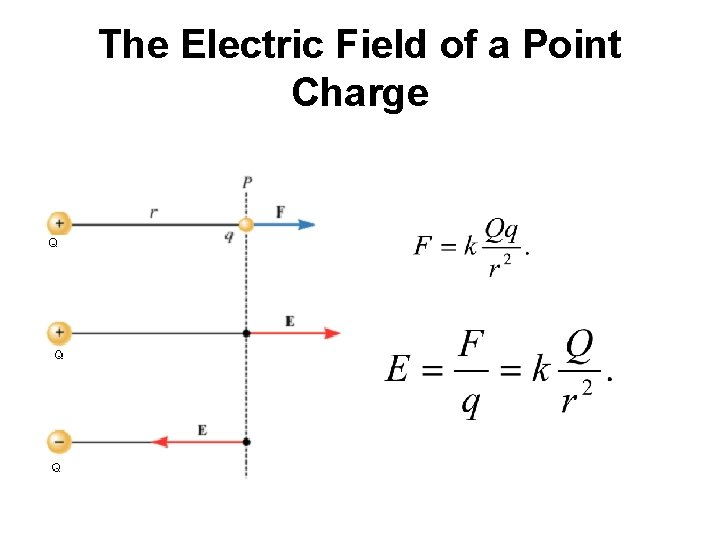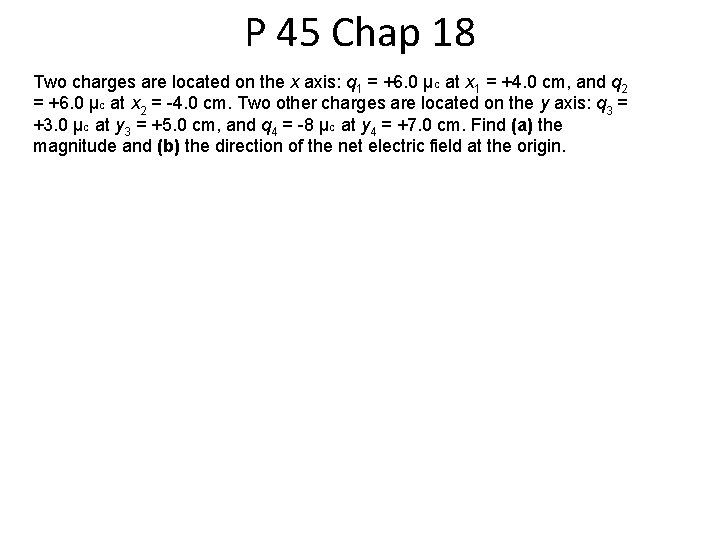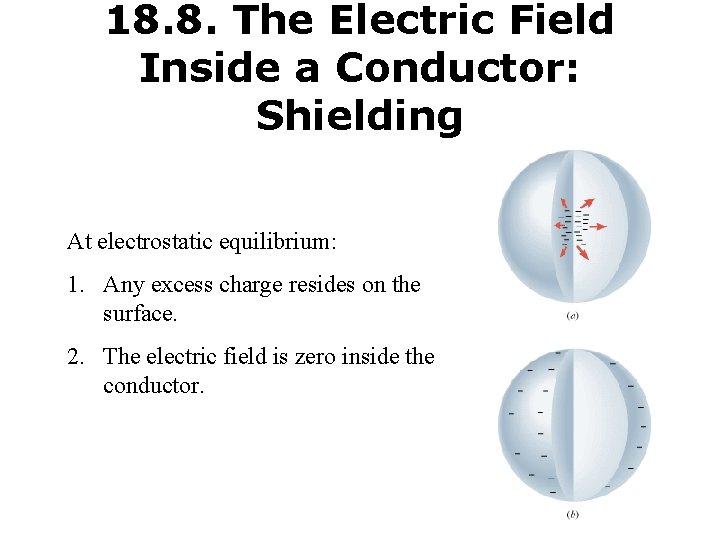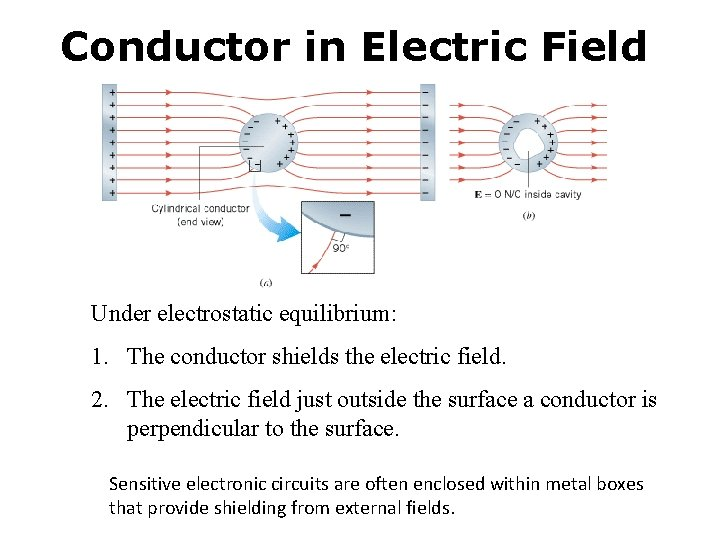The Electric Field The electric field is present

• Slides: 9The Electric Field The electric field is present in any region of space if there exists electric forces on charges. These electric forces can be detected using a test charge. Test charges are theoretical positive charges that do not alter the electric field to be detected. Electric field at a point in space is defined as the electric force per unit test charge placed at that point. The SI unit for the electric field is the newton per coulomb (N/C). Electric field is a vector.Electric ForceThe Electric Field of a Point ChargeP 45 Chap 18 Two charges are located on the x axis: q 1 = +6. 0 µC at x 1 = +4. 0 cm, and q 2 = +6. 0 µC at x 2 = -4. 0 cm. Two other charges are located on the y axis: q 3 = +3. 0 µC at y 3 = +5. 0 cm, and q 4 = -8 µC at y 4 = +7. 0 cm. Find (a) the magnitude and (b) the direction of the net electric field at the origin.Electric Field Lines • • • Positive point charge Negative point charge Dipole Two like charges Parallel plate CapacitorGauss' Law: The electric flux, ΦE through a Gaussian surface is equal to the net charge Q enclosed by the surface divided by, ϵ 0 the permittivity of free space:18. 8. The Electric Field Inside a Conductor: Shielding At electrostatic equilibrium: 1. Any excess charge resides on the surface. 2. The electric field is zero inside the conductor.Conductor in Electric Field Under electrostatic equilibrium: 1. The conductor shields the electric field. 2. The electric field just outside the surface a conductor is perpendicular to the surface. Sensitive electronic circuits are often enclosed within metal boxes that provide shielding from external fields.18. 10 Copying Machine